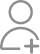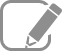Take Class 12 Tuition from the Best Tutors

•Affordable fees
•1-1 or Group class
•Flexible Timings
•Verified Tutors

Search in

# The amplitude of the magnetic field part of a harmonic electromagnetic wave in vacuum is B0 = 510 nT. What is the amplitude of the electric field part of the wave?

Learn Exercise 8Follow 1AnswerAmplitude of magnetic field of an electromagnetic wave in a vacuum, B0 = 510 nT = 510 × 10−9 T Speed of light in a vacuum, c = 3 × 108 m/s Amplitude of electric field of the electromagnetic wave is given by the relation, E = cB0 = 3 × 108 × 510 × 10−9 = 153 N/C Therefore,...

Amplitude of magnetic field of an electromagnetic wave in a vacuum,

B0 = 510 nT = 510 × 10−9 T

Speed of light in a vacuum, c = 3 × 108 m/s

Amplitude of electric field of the electromagnetic wave is given by the relation,

E = cB0

= 3 × 108 × 510 × 10−9 = 153 N/C

Therefore, the electric field part of the wave is 153 N/C.

Now ask question in any of the 1000+ Categories, and get Answers from Tutors and Trainers on UrbanPro.com

Find Grade 12 Tuition near you

Looking for Class 12 Tuition ?

Learn from the Best Tutors on UrbanPro

Are you a Tutor or Training Institute?

Join UrbanPro Today to find students near you
X

### Looking for Class 12 Tuition Classes?

The best tutors for Class 12 Tuition Classes are on UrbanPro

• Select the best Tutor
• Book & Attend a Free Demo
• Pay and start Learning### Take Class 12 Tuition with the Best Tutors

The best Tutors for Class 12 Tuition Classes are on UrbanPro